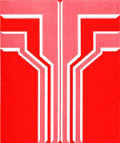1972

#### Citation

Published in Transactions of the Nebraska Academy of Sciences, Volume 1 (1972).

#### Abstract

The method of finite integral transforms (Eringen, 1954; Churchill, 1955; Sneddon, 1955; Cinelli, 1965; Roettinger, 1947) has gradually replaced the classical method of separation of variables. This is undoubtedly due to its compact and almost drill-like formalism. Wherever applicable, it has also replaced the Laplace transform with the resulting avoidance of cumbersome contour integrals in the inversion.

In this paper, we are concerned with solving, by such a transformational or operational method, the following general boundary-value problem:

Li (fi) + M (fi) = gi (x), Ci-l

a1f1(Co) - a2f1(C0) = D1

a3fn(Cn) + a4f n(Cn) = D2

A1jf 'j(C-j) = A1(j+1) f'j+1 (C+j)

where

i= 1, 2, 3,.... n

j= 1, 2, 3, ...., (n-1)

and M is certain linear differential operator involving independent variables other than x, with associated linear conditions not exhibited. gi(x) are given bounded functions of x, which may depend on the other independent variables. D1 and D2 are given functions of independent variables other than x. a1, a2, a3, a4, (a1 and a2 are not both zero, a3 and a4 are not both zero) Ali, A2i, A3i, Hj and aj are all given constants; a1, a3 and aj being either 1 of 0. PI (x), P2(X) and P3(x) are given continuous functions. The prime represents here differentiation with respect to x. If PI (Co) = 0, condition (2) is to be replaced by

COinS